We've learned two really important things in recent chapters: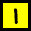How to solve a quadratic = 0 :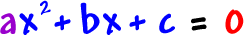How to graph a quadratic: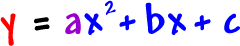Now, let's make the connection between the two!  I'll explain it with an example:

Let's graph this guy: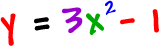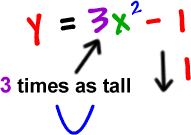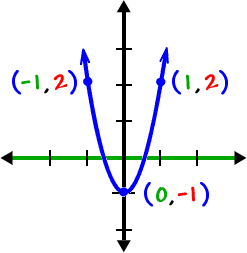But, there's one other really important thing we need to know:

Where does this guy cross the x-axis?

From our graph, it LOOKS like it might be around x = - .6 and x = .6 ...  How can we be sure?